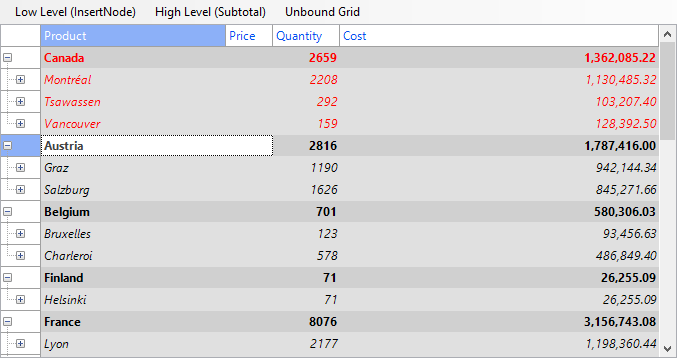﻿ Creating Outlines and Trees in Unbound Grids# Creating Outlines and Trees in Unbound Grids

Earlier we introduced creation of outlines and trees with bound C1FlexGrid control. This time we will look how to create outline tree in unbound grid and will use some features that cannot be used in bound scenario.

Let's start with typical grid showing product, country, city, and sales amounts: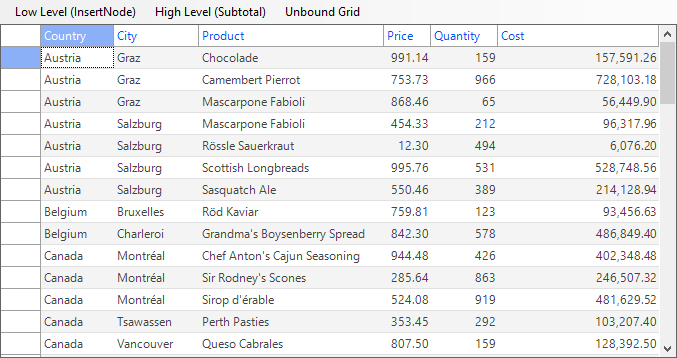For populating grid with data we will create special class with constants of information:

``````
public static class Consts
{
public const int maxRecordCount = 2000;

public static readonly Dictionary countriesAndCities = new Dictionary()
{
{"Argentina", new string[] {"Buenos Aires"}},
{"Austria", new string[] {"Graz", "Salzburg"}},
{"Belgium", new string[] {"Bruxelles", "Charleroi"}},
{"Brazil", new string[] {"Campinas", "Resende", "Rio de Janeiro", "Sao Paulo"}},
{"Canada", new string[] {"Montréal", "Tsawassen", "Vancouver"}},
{"Denmark", new string[] {"Århus", "Kobenhavn"}},
{"Finland", new string[] {"Helsinki", "Oulu"}},
{"France", new string[] {"Lille", "Lyon", "Marseille", "Nantes", "Paris", "Reims", "Strasbourg", "Toulouse", "Versailles"}},
{"Germany", new string[] {"Aachen", "Berlin", "Brandenburg", "Cunewalde", "Frankfurt a.M.", "Köln", "Leipzig", "Mannheim", "München", "Münster", "Stuttgart"}},
{"Ireland", new string[] {"Cork"}},
{"Italy", new string[] {"Bergamo", "Reggio Emilia", "Torino"}},
{"Mexico", new string[] {"México D.F."}},
{"Norway", new string[] {"Stavern"}},
{"Poland", new string[] {"Warszawa"}},
{"Portugal", new string[] {"Lisboa"}},
{"Spain", new string[] {"Barcelona", "Madrid", "Sevilla"}},
{"Sweden", new string[] {"Bräcke", "Luleå"}},
{"Switzerland", new string[] {"Bern", "Genève"}},
{"UK", new string[] {"Colchester", "Cowes", "London"}},
{"USA", new string[] { "Albuquerque", "Anchorage", "Boise", "Butte", "Elgin", "Eugene", "Kirkland", "Lander", "Portland", "San Francisco", "Seattle", "Walla Walla"}},
{"Venezuela", new string[] {"Barquisimeto", "Caracas", "I. de Margarita", "San Cristóbal"}}
};

public static readonly string[] products = new string[]
{
"Alice Mutton", "Aniseed Syrup", "Boston Crab Meat",
"Camembert Pierrot", "Carnarvon Tigers", "Chai",
"Chang", "Chartreuse verte", "Chef Anton's Cajun Seasoning",
"Chef Anton's Gumbo Mix", "Chocolade", "Côte de Blaye",
"Escargots de Bourgogne", "Filo Mix", "Flotemysost",
"Geitost", "Genen Shouyu", "Gnocchi di nonna Alice",
"Guaraná Fantástica", "Gudbrandsdalsost", "Gula Malacca",
"Gumbär Gummibärchen", "Gustaf's Knäckebröd", "Ikura",
"Inlagd Sill", "Ipoh Coffee", "Jack's New England Clam Chowder",
"Konbu", "Lakkalikööri", "Laughing Lumberjack Lager",
"Longlife Tofu", "Louisiana Fiery Hot Pepper Sauce", "Louisiana Hot Spiced Okra",
"Manjimup Dried Apples", "Mascarpone Fabioli", "Maxilaku",
"Mishi Kobe Niku", "Mozzarella di Giovanni", "Nord-Ost Matjeshering",
"Northwoods Cranberry Sauce", "NuNuCa Nuß-Nougat-Creme", "Original Frankfurter grüne Soße",
"Outback Lager", "Pâté chinois", "Pavlova",
"Perth Pasties", "Queso Cabrales", "Queso Manchego La Pastora",
"Raclette Courdavault", "Ravioli Angelo", "Rhönbräu Klosterbier",
"Röd Kaviar", "Rogede sild", "Rössle Sauerkraut",
"Singaporean Hokkien Fried Mee", "Sir Rodney's Marmalade", "Sir Rodney's Scones",
"Sirop d'érable", "Spegesild", "Steeleye Stout",
"Tarte au sucre", "Teatime Chocolate Biscuits", "Thüringer Rostbratwurst",
"Tofu", "Tourtière", "Tunnbröd",
"Uncle Bob's Organic Dried Pears", "Valkoinen suklaa", "Vegie-spread",
"Wimmers gute Semmelknödel", "Zaanse koeken"
};
}

``````

Before populating the grid we should setup it in the proper way - add enough columns for data, add fixed columns and rows for headers, assign proper DataType for each column:

``````
void SetupGrid()
{
// prepare grid for filling with data

// start from first non-fixed column for setup grid
int columnIndex = _flex.Cols.Fixed;
_flex.Cols[columnIndex].DataType = typeof(string);
_flex.Cols[columnIndex].Caption = "Country";
_flex.Cols[columnIndex].Name = "Country";

columnIndex++;
_flex.Cols[columnIndex].DataType = typeof(string);
_flex.Cols[columnIndex].Caption = "City";
_flex.Cols[columnIndex].Name = "City";

columnIndex++;
_flex.Cols[columnIndex].DataType = typeof(string);
_flex.Cols[columnIndex].Caption = "Product";
_flex.Cols[columnIndex].Name = "Product";

columnIndex++;
_flex.Cols[columnIndex].DataType = typeof(decimal);
_flex.Cols[columnIndex].Caption = "Price";
_flex.Cols[columnIndex].Name = "Price";

columnIndex++;
_flex.Cols[columnIndex].DataType = typeof(short);
_flex.Cols[columnIndex].Caption = "Quantity";
_flex.Cols[columnIndex].Name = "Quantity";

columnIndex++;
_flex.Cols[columnIndex].DataType = typeof(decimal);
_flex.Cols[columnIndex].Caption = "Cost";
_flex.Cols[columnIndex].Name = "Cost";
_flex.Cols[columnIndex].AllowEditing = false;

// format Price column
_flex.Cols["Price"].Format = "n2";

// format Cost column
_flex.Cols["Cost"].Format = "n2";

// allow node content to spill onto next cell
_flex.AllowMerging = AllowMergingEnum.Nodes;
}

``````

After this step our grid will look like this: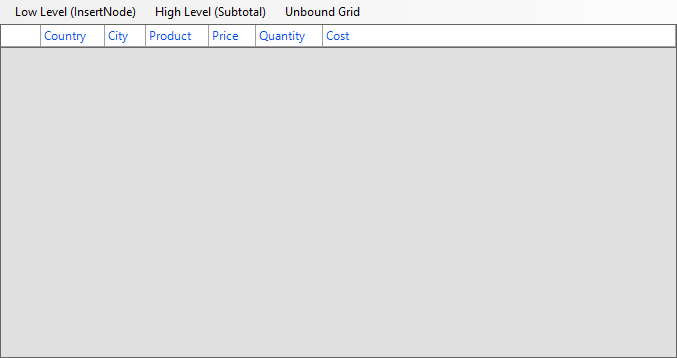Now we will generate data for the grid. This data will be used to fill grid in unbound mode:

``````
void GenerateDataRows()
{
// fill only if data does not exist
if (dataRows.Count == 0)
{
// generate rows with random data for grid
Random random = new Random();
int currentRecordIndex = \_flex.Rows.Count - \_flex.Rows.Fixed;
int currentCountryIndex = 0;
int currentCityIndex = 0;

while (currentRecordIndex < Consts.maxRecordCount &&
(currentCountryIndex != Consts.countriesAndCities.Count - 1 ||
(currentCountryIndex == Consts.countriesAndCities.Count - 1 &&
currentCityIndex != Consts.countriesAndCities.Last().Value.Length - 1)))
{
bool useCurrentCity = random.Next(0, 2) == 1;

// use current city
if (useCurrentCity)
{
object[] dataRow = new object;
dataRow = Consts.countriesAndCities.ElementAt(currentCountryIndex).Key;
dataRow = Consts.countriesAndCities.ElementAt(currentCountryIndex).Value[currentCityIndex];
dataRow = Consts.products[random.Next(0, Consts.products.Length)];

// generate price
Decimal price = Convert.ToDecimal(Convert.ToSingle(random.Next(0, 100000)) / 100);
dataRow = price;

// generate quantity
short quantity = Convert.ToInt16(random.Next(0, 1000));
dataRow = quantity;

// calculate cost
dataRow = Convert.ToDecimal(price * quantity);
}

// use next city
else if (currentCityIndex < Consts.countriesAndCities.ElementAt(currentCountryIndex).Value.Length - 1)
currentCityIndex++;

// use next country
else
{
currentCountryIndex++;
currentCityIndex = 0;
}

// move to next row of data
currentRecordIndex++;
}
}
}

``````

The code will generate sorted data for “Country” and “City” columns. This is required to prevent extra sort operations. We can fill our grid with generated data this way:

``````
void PopulateGrid()
{
// populate grid with generated data
for (int i = 0; i < dataRows.Count; i++)
{
// handle fixed columns
if (dataRows[i].Length < _flex.Cols.Count)
{
object[] tmpRow = dataRows[i];
dataRows[i] = new object[_flex.Cols.Count];
dataRows[i] = null;
Array.Copy(tmpRow, 0, dataRows[i], 1, tmpRow.Length);
}
if (dataRows[i].Length > _flex.Cols.Count)
{
object[] tmpRow = dataRows[i];
dataRows[i] = new object[_flex.Cols.Count];
Array.Copy(tmpRow, 1, dataRows[i], 0, dataRows[i].Length);
}
}
}

``````

To keep the data in the grid always updated we will handle CellChanged event of C1FlexGrid:

``````
private void \_flex\_CellChanged(object sender, RowColEventArgs e)
{
// check in what column data was changed
if (\_flex.Cols[e.Col].Name == "Price" || \_flex.Cols[e.Col].Name == "Quantity")
{
// check data
if (Convert.ToInt32(_flex[e.Row, e.Col]) < 0)
_flex[e.Row, e.Col] = 0;

// calculate new cost
if (\_flex[e.Row, "Price"] != null && \_flex[e.Row, "Quantity"] != null)
\_flex[e.Row, "Cost"] = (decimal)\_flex[e.Row, "Price"] * (short)_flex[e.Row, "Quantity"];
}
}

``````

## Outline Tree

The outline tree is very similar to the one you see in a regular TreeView control. It shows an indented structure with collapse/expand icons next to each node row so that user can expand and collapse the outline to see the desired level of details. The outline tree can be displayed in any column, defined by the Tree.Column property. By default, this property is set to -1, which causes the tree not to be displayed at all. To show the outline tree in the example given above, use this code:

``````
// group on a given column inserting nodes of a given level
void GroupBy(string columnName, int level)
{
object current = null;
for (int r = \_flex.Rows.Fixed; r < \_flex.Rows.Count; r)
{
if (!_flex.Rows[r].IsNode)
{
var value = _flex[r, columnName];
if (!object.Equals(value, current))
{
// value changed: insert node
_flex.Rows.InsertNode(r, level);

// show group name in first scrollable column
\_flex[r, \_flex.Cols.Fixed] = value;

// update current value
current = value;
}
}
}
}

void \_btnTreeCountryCity\_Click(object sender, EventArgs e)
{
using (new DeferRefresh(_flex))
{
// group by country and city as before
GroupBy("Country", 0);
GroupBy("City", 1);

// show outline tree
_flex.Tree.Column = 0;

// autosize to accommodate tree
\_flex.AutoSizeCol(\_flex.Tree.Column);

// collapse detail nodes
_flex.Tree.Show(1);
}
}

``````

The GroupBy method shows how to insert node rows grouping identical values on a given column. The remaining code calls this method to build the outline, and then sets the Tree.Column property to zero in order to show the outline tree in the first column. It also calls the AutoSizeCol.html) method to ensure that the column is wide enough to accommodate the outline tree. Finally, it calls the Tree.Show method to display all level-0 nodes (cities in this case) and hide all the details. To make the outlines really useful, the node rows should include summary information for the data they contain. Since we created the outline tree using the Rows.InsertNode.html) method, we'll use the Aggregate.html) method to calculate the subtotals for each group of rows and insert the result directly into the node rows. The AddSubtotals method listed below shows how to do this:

``````
// add subtotals to each node at a given level
{
// get column we are going to total on
int colIndex = _flex.Cols.IndexOf(colName);

// scan rows looking for nodes at the right level
for (int r = \_flex.Rows.Fixed; r < \_flex.Rows.Count; r)
{
if (_flex.Rows[r].IsNode)
{
var node = _flex.Rows[r].Node;
if (node.Level == level)
{
// found a node, calculate the sum of extended price
var range = node.GetCellRange();
var sum = _flex.Aggregate(AggregateEnum.Sum,
range.r1, colIndex, range.r2, colIndex,
AggregateFlags.ExcludeNodes);

// show the sum on the grid
// (will use the column format automatically)
_flex[r, colIndex] = sum;
}
}
}
}

``````

The AddSubtotals method scans the grid rows looking for node rows. When a node row of the desired level is found, the method uses the Node.GetCellRange method to retrieve the node's child rows. Then it uses the Aggregate.html) method to calculate the sum of the values on the target column over the entire range. The call to Aggregate.html) includes the ExcludeNodes flag to avoid double-counting existing nodes. Once the subtotal has been calculated, it is assigned to the node row's cell with the usual _flex[row, col] indexer. This method can be used to add multiple totals to each node row. In this example, we'll add totals for the Quantity and Cost columns. In addition to sums, you could add other aggregates such as average, maximum, minimum, etc. We can use this method to create a complete outline, with node rows, outline tree, and subtotals:

``````
void \_btnTreeCountryCity\_Click(object sender, EventArgs e)
{
using (new DeferRefresh(_flex))
{
// restore original sort (by Country, City, SalesPerson)
ResetData();

// group by Country, City
GroupBy("Country", 0); // group by country (level 0)
GroupBy("City", 1); // group by city (level 1)

// add totals per Country, City
AddSubtotals(0, "Cost"); // cost per country (level 0)
AddSubtotals(0, "Quantity"); // quantity per country (level 0)
AddSubtotals(1, "Cost"); // cost per city (level 1)
AddSubtotals(1, "Quantity"); // quantity per city (level 1)

// show outline tree
_flex.Tree.Column = 0;
\_flex.AutoSizeCol(\_flex.Tree.Column);
_flex.Tree.Show(1);
}
}

``````

If you run the project now, you'll see a tree with node rows that show the total quantity and amount sold for each country and city. This is very nice, but if you expand any of the node rows, you'll see a lot of duplicate values. All rows under a given city node have the same country and city: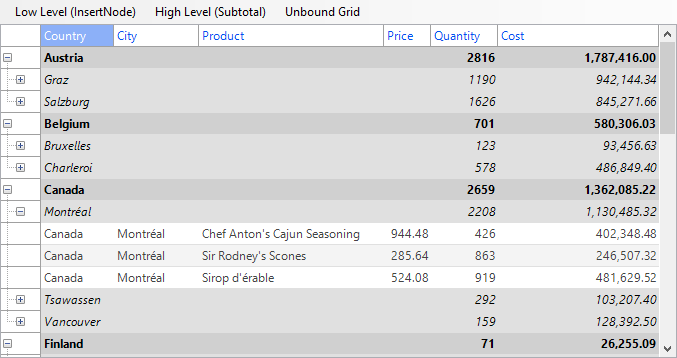This is correct, but it's a waste of a screen space. Eliminating these duplicate values is easy: just set the Width of the columns that are being grouped on to zero. When you do that, remember to set the grid's AllowMerging property to Nodes, so the text assigned to the node rows will spill into the visible columns. (Another option would be to assign the node text to the first visible column, but merging is usually a better solution because it allows you to use longer text for the node rows). Here's the revised code and the final result:

``````
void \_btnTreeCountryCity\_Click(object sender, EventArgs e)
{
using (new DeferRefresh(_flex))
{
// restore original sort (by Country, City, SalesPerson)
ResetData();

// group by Country, City
GroupBy("Country", 0); // group by country (level 0)
GroupBy("City", 1); // group by city (level 1)

// hide columns that we grouped on
// (they only have duplicate values which already appear on the tree nodes)
// (but don't make them invisible, that would also hide the node text)
_flex.Cols["Country"].Width = 0;
_flex.Cols["City"].Width = 0;

// allow node content to spill onto next cell
_flex.AllowMerging = AllowMergingEnum.Nodes;

// add totals per Country, City
AddTotals(0, "Cost"); // cost per country (level 0)
AddTotals(0, "Quantity"); // quantity per country (level 0)
AddTotals(1, "Cost"); // cost per city (level 1)
AddTotals(1, "Quantity"); // quantity per city (level 1)

// show outline tree
_flex.Tree.Column = 0;
\_flex.AutoSizeCol(\_flex.Tree.Column);
_flex.Tree.Show(1);
}
}

``````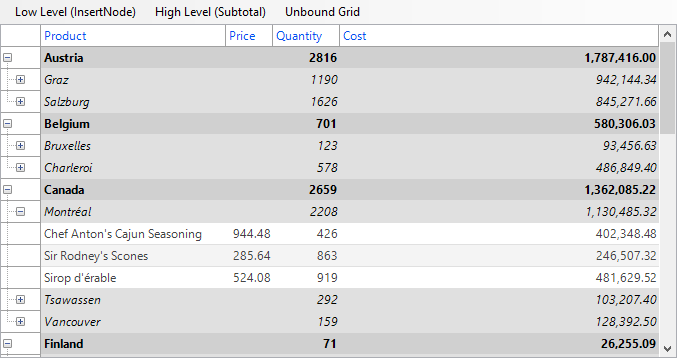The Country and City columns are now invisible, but their values still appear in the node rows. Collapsing the tree shows totals for each country and city. Another option to create trees is using the C1FlexGrid's Subtotal.html).html).html) method. The Subtotal.html).html).html) method performs the same tasks as the GroupBy and AddSubtotals methods described above, except it does both things in a single step and is therefore a little more efficient. The code below shows how you can use the Subtotal.html).html).html) method to accomplish the same thing we did before, only a little faster and without using any helper methods:

``````
void \_btnTreeCountryCity\_Click(object sender, EventArgs e)
{
using (new DeferRefresh(_flex))
{
// restore original sort (by Country, City, SalesPerson)
ResetData();

// group and total by country and city
_flex.Subtotal(AggregateEnum.Sum, 0, "Country", "Cost");
_flex.Subtotal(AggregateEnum.Sum, 0, "Country", "Quantity");
_flex.Subtotal(AggregateEnum.Sum, 1, "City", "Cost");
_flex.Subtotal(AggregateEnum.Sum, 1, "City", "Quantity");

// hide columns that we grouped on
// (they only have duplicate values which already appear on the tree nodes)
// (but don't make them invisible, that would also hide the node text)
_flex.Cols["Country"].Width = 0;
_flex.Cols["City"].Width = 0;
_flex.AllowMerging = AllowMergingEnum.Nodes;

// show outline tree
_flex.Tree.Column = 0;
\_flex.AutoSizeCol(\_flex.Tree.Column);
_flex.Tree.Show(1);
}
}

``````

The Subtotal method is very convenient and flexible. It has a number of overloads that allow you to specify which columns should be grouped on and totaled on by index or by name, whether to include a caption in the node rows that it inserts, how to perform the grouping, and so on. The both techniques that we used to create outline trees are the same as for bound scenario.

## Using the Node class

In unbound grid we can use some features that cannot be used in bound mode. For example any row can be turned to node simply by setting its IsNode property to true:

``````
_flex.Rows[index].IsNode = true;

``````

Also we can use Node.Move.html) method to move nodes across the grid. For our example we will add feature that will lock selected top-level node on the top of the grid:

``````
void MoveSelectedTopNodeToGridTop()
{
Node nodeToMove = null;

if (\_flex.Rows[\_flex.Row].IsNode)
nodeToMove = \_flex.Rows[\_flex.Row].Node;
else
{
// current selected row
int rowIndex = _flex.Row;

// search node above row with data or below
int increment = _flex.SubtotalPosition == SubtotalPositionEnum.AboveData ? -1 : 1;

// search deepest node
while (rowIndex > _flex.Rows.Fixed)
{
if (_flex.Rows[rowIndex].IsNode)
{
nodeToMove = _flex.Rows[rowIndex].Node;
break;
}
rowIndex += increment;
}
}

if (nodeToMove != null)
{
while (nodeToMove.Level != 0)
nodeToMove = nodeToMove.Parent;

// restoring position of previous moved node
if (_movedTopNodeRowIndex != -1)
{
// getting all cells of previous moved top-level node
CellRange movedTopNodeRange = \_flex.Rows[\_movedTopNodeRowIndex].Node.GetCellRange();

// move node with all its children to initial position
int rowsCount = movedTopNodeRange.BottomRow - movedTopNodeRange.TopRow + 1;
if (_lastPreviousRowIndex != -1)
\_flex.Rows.MoveRange(movedTopNodeRange.r1, rowsCount, \_lastPreviousRowIndex - rowsCount + 1);
else if (_lastNextRowIndex != -1)
\_flex.Rows.MoveRange(movedTopNodeRange.r1, rowsCount, \_lastNextRowIndex - rowsCount + 1);
}

// find previous and next node positions for reverting position previous moved node
Node prevNode = nodeToMove.PrevNode;
Node nextNode = nodeToMove.NextNode;

// moving node to top of the grid
nodeToMove.Move(NodeMoveEnum.First);

// store indexes of previous, next and moved rows
if (prevNode != null)
{
CellRange cr = prevNode.GetCellRange();
_lastPreviousRowIndex = cr.BottomRow;
}
else
_lastPreviousRowIndex = -1;

if (nextNode != null)
_lastNextRowIndex = nextNode.Row.Index - 1;
else
_lastNextRowIndex = -1;

_movedTopNodeRowIndex = nodeToMove.Row.Index;
_flex.TopRow = nodeToMove.Row.Index;

// getting all cells of just moved top-level node
CellRange nodeToMoveRange = nodeToMove.GetCellRange();
}
}

``````

Now we can select any child of any top-level node and move this node to the top of the grid: